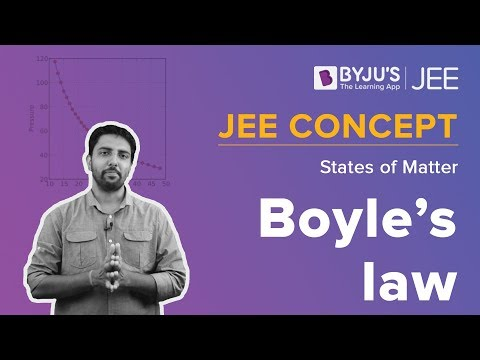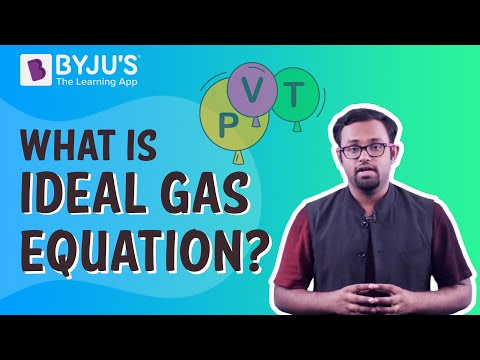# Boyles Law Questions

Boyle’s law is also referred to as Boyle–Mariotte law or Mariotte’s law. It tells us about the behaviour of gases. Boyle’s law states that the pressure is inversely proportional to the volume of the gas at constant pressure.

P ∝ 1 / V

Or, PV = k

 Definition: Boyle’s law states that at a constant temperature, the pressure of a gas is inversely proportional to its volume.

## Boyles Law Chemistry Questions with Solutions

Q1. Suppose P, V, and T represent the gas’s pressure, volume, and temperature, then the correct representation of Boyle’s law is

1. V is inversely proportional to T (at constant P)
2. V inversely proportional to P (at constant T)
3. PV = nRT
4. PV = RT

Answer: (b), If P, V, and T represent the gas’s pressure, volume, and temperature, then the correct representation of Boyle’s law is V inversely proportional to P (at constant T).

V ∝ 1 / P

Q2. What is the nature of Boyle’s Law’s pressure vs volume (P vs V) graph?

1. Straight Line
2. Rectangular Hyperbola
3. Parabola
4. None of the above

Answer: (b), The nature of Boyle’s Law’s pressure vs volume (P vs V) graph is a rectangular hyperbola.

Q3. What is the nature of Boyle’s Law’s pressure-volume vs pressure (PV vs P) graph?

1. Straight-line parallel to the P axis
2. Straight-line parallel to the PV axis
3. Straight-line parallel to the V axis
4. None of the above

Answer: (a), The nature of Boyle’s Law’s pressure-volume vs pressure (PV vs P) graph is a straight line parallel to the P axis.

Q4. Which of the following quantity is kept constant in Boyle’s law?

1. Gas mass only
2. Gas Temperature only
3. Gas Mass and Gas Pressure
4. Gas Mass and Gas Temperature

Answer: (d), In Boyle’s law, the mass of the gas its temperature are kept constant.

Q5. Boyle’s law is valid only for

1. Ideal gases
2. Non-ideal gases
3. Light Gases
4. Heavy Gases

Answer: (a), Boyle’s law is valid only for ideal gases.

Q6. What is Boyle’s law?

Answer: Boyle’s law depicts the relationship between the pressure, volume, and temperature of a gas. It states that the pressure of a gas is inversely proportional to its volume at a constant temperature.

P ∝ 1 / V

Or, PV = k

Q7. How is Boyle’s law used in everyday life?

Answer: Boyle’s law can be observed in our everyday life. Filling air in the bike tire is one of the significant applications of Boyle’s law. While pumping air into the tyre, the gas molecules inside the tire are compressed and packed closer together. It increases the pressure exerted on the walls of the tyre.

Q8. What is Boyle’s temperature?

Answer: Boyle’s temperature is the temperature at which the real and non-ideal gases behave like an ideal gas over a broad spectrum of pressure. It is related to the Van der Waal’s constant a, b as TB = a / Rb

Q9. Differentiate between Boyle’s law and Charle’s law.

S. No.

Boyle’s Law

Charle’s Law

1.

Boyle’s law gives a relation between the pressure and the volume of the gas.

Charle’s law gives a relation between the temperature and the volume of the gas.

2.

Temperature is kept constant.

Pressure is kept constant.

3.

Pressure is inversely proportional to the volume.

Temperature is directly proportional to the volume.

4.

P ∝ 1 / V

T ∝ V

5.

The product of the pressure and the volume is constant.

The ratio of the temperature and the volume is constant.

6.

PV = k

V = kT

Q10. Match the following gas laws with the equation representing them.

Column 1

Column 2

Boyle’s law

PV = nRT

Charles’ law

V = kN at constant temperature and pressure

Dalton’s law

PTOTAL = P1 + P2 + P3 + P4 + . . . Pat constant temperature and volume

V = kT at a constant pressure

Ideal Gas law

PV = k at a constant temperature

Column 1

Column 2

Boyle’s law

PV = k at a constant temperature

Charles’ law

V = kT at a constant pressure

Dalton’s law

PTOTAL = P1 + P2 + P3 + P4 + . . . Pat constant temperature and volume

V = kN at constant temperature and pressure

Ideal Gas law

PV = nRT

Q11. A helium balloon has a volume of 735 mL at ground level. The balloon is transported to an elevation of 5 km, where the pressure is 0.8 atm. At this altitude, the gas occupies a volume of 1286 mL. Assuming that the temperature is constant, what was the ground level pressure?

Initial Volume (V1 ) = 735 mL

Final Pressure (P2 ) = 0.8 atm

Final Volume (V2 ) = 1286 mL

To Find: Initial Pressure (P1 ) = ?

We can calculate the initial pressure of the gas using Boyle’s law.

P1 V1 = P2 V2

P 1 X 735 = 0.8 X 1286

P1 = 1028.8 / 735

P1 = 1.39 ≈ 1.4 atm

Hence the ground level pressure is 1.4 atm.

Q12. A sample of oxygen gas has a volume of 225 mL when its pressure is 1.12 atm. What will the volume of the gas be at a pressure of 0.98 atm if the temperature remains constant?

Initial Volume (V1 ) = 225 mL

Initial Pressure (P1 ) = 1.12 atm

Final Pressure (P2 ) = 0.98 atm

To Find: Final Volume (V 2 ) = ?

We can calculate the final volume of the gas using Boyle’s law.

P1 V1 = P2 V2

1.12 X 225 = 0.98 X V 2

252 = 0.98 X V 2

252 / 0.98 = V 2

V 2 = 257.14 mL ≈ 257mL

Hence the final volume of the gas at pressure of 0.98 atm is equivalent to 257 mL.

Q13. An ideal gas occupying a 2.0 L flask at 760 torrs is allowed to expand to a volume of 6,000 mL. Calculate the final pressure

Initial Volume (V1 ) = 2 L

Initial Pressure (P1 ) = 760 torrs

Final Volume (V2 ) = 6000 mL = 6 L

To Find: Final Pressure (P 2 ) = ?

We can calculate the final pressure of the gas using Boyle’s law.

P1 V1 = P2 V2

760 X 2 = P2 X 6

1520 = P2 X 6

P2 = 1520 / 6

P2 = 253.33 torrs ≈ 253 torrs

Hence the final pressure of the gas at volume of 6 L is equivalent to 253 torrs.

Q14. A gas occupies a volume of 1 L and exerts a pressure of 400 kPa on the walls of its container. What would be the pressure exerted by the gas if it is completely transferred into a new container having a volume of 3 litres (assuming that the temperature and amount of the gas remain the same.)?

Initial Volume (V1 ) = 1 L

Initial Pressure (P1 ) = 400 kPa

Final Volume (V2 ) = 3 L

To Find: Final Pressure (P 2 ) = ?

We can calculate the final pressure of the gas using Boyle’s law.

P1 V1 = P2 V2

400 X 1 = P 2 X 3

P 2 = 400 / 3

P 2 = 133.33 ≈ 133 kPa

Hence the final pressure of the gas at of volume 3 L is equivalent to 133 kPa.

Q15. A gas exerts a pressure of 3 kPa on the walls of container 1. When container one is emptied into a 10 litre container, the pressure exerted by the gas increases to 6 kPa. Find the volume of container 1. Assume that the temperature and amount of the gas remain the same.

Initial Pressure (P1 ) = 3 kPa

Final Volume (V2 ) = 10 L

Final Pressure (P2 ) = 6 kPa

To Find: Initial Volume (V 1 ) = ?

We can calculate the initial volume of the gas using Boyle’s law.

P1 V1 = P2 V2

3 X V 1 = 6 X 10

3 X V 1 = 60

V 1 = 60 / 3

V 1 = 20 L

Hence the initial volume of the gas at pressure of 3 kPa is equivalent to 20 L.

## Practise Questions on Boyle’s Law

Q1. A gas is initially in a 5 L piston with a pressure of 1 atm. What is the new volume if the pressure changes to 3.5 atm by moving the piston down?

Q2. A balloon of volume 0.666 L at 1.03atm is placed in a pressure chamber where the pressure becomes 5.68atm. Determine the new volume.

Q3. A gas in a 30.0 mL container is at a pressure of 1.05 atm and is compressed to a volume of 15.0 mL. What is the new pressure of the container?

Q4. If a gas occupies 3.60 litres at a pressure of 1.00 atm, what will be its volume at a pressure of 2.50 atm?

Q5. A gas occupies 12.3 litres at a pressure of 40.0 mmHg. What is the volume when the pressure is increased to 60.0 mmHg?

Click the PDF to check the answers for Practice Questions.

## Recommended Videos

#### Boyle’s Law#### Ideal Gas Equation definitions, derivation